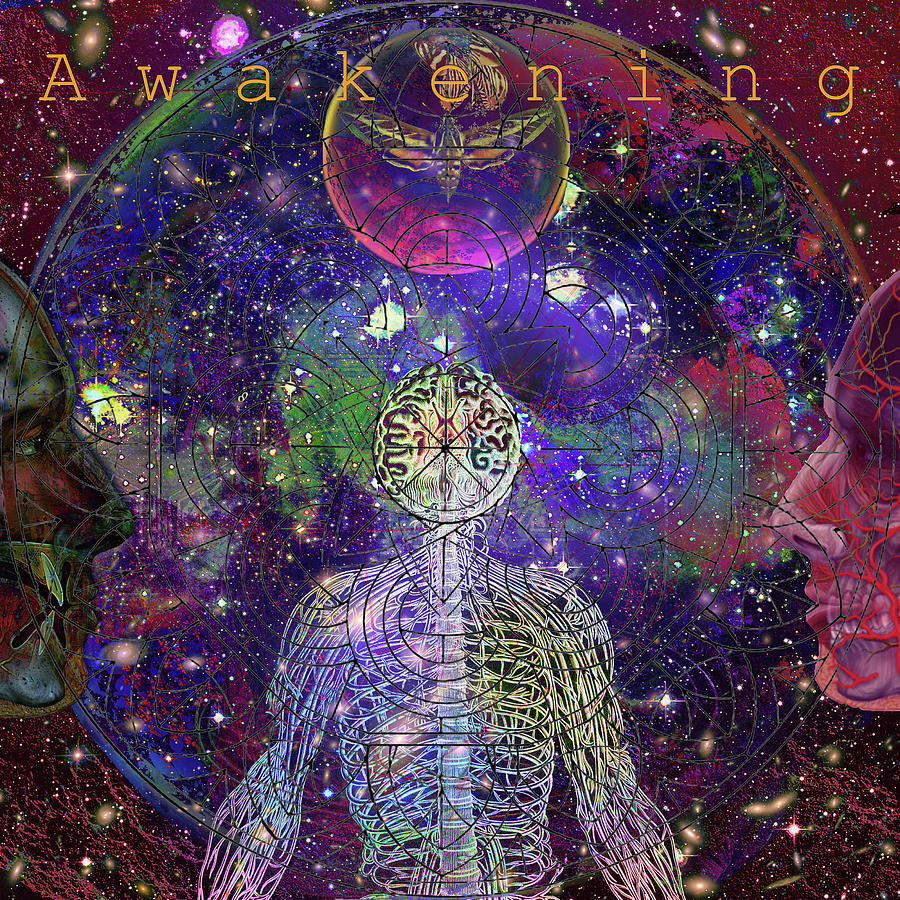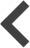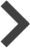A W A K E N I N G Solarl I F EPewaukee, WI - United States

The Artist Who Was Formerly Known As SixFONT ART. LF"Feature";-)Thank"U"J";-)

Tallahassee, FL - United States

Once known as a Cosmic Revelation in the stoner community. Joseph, thank you for posting this work to FONT ART. LF

Pewaukee, WI - United States

Bob & Nadine Johnston " All Media Acceptable Group f e a t u r e...Thank'U" Bob & Nadine ;-)

Pewaukee, WI - United States

F i n e A r t s P r o f e s s i o n a l G r o u p f e a t u r e...N a g e l R a n g i d a n ;-)ThankYOU!

Pewaukee, WI - United States

F i n e A r t s P r o f e s s i o n a l G r o u p f e a t u r e...N a g e l R a n g i d a n ;-)ThankYOU!

Pewaukee, WI - United States

A l o n g T h e T r a i l" T h e S o c i a l A r t s G r o u p F e a t u r e...THANKyOU! A.T.T. ;-)

Pewaukee, WI - United States

J e n n P o i n t e r "F i g u r a t i v e A b s t r a c t i o n G r o u p F e a t u r e" T H A N KyO U! J e n n;-)

Pewaukee, WI - United States

Michelle Reid" C o l l e c t o r s G a l l e r y G r o u p F e a t u r e...T h a n k y o u M s. R e i d ;-)

Pewaukee, WI - United States

Thks!Genevieve;-)

Pewaukee, WI - United States

Genevieve Esson" L y r i c a l T r e e s - V i b r a n t W a v e l e n g h O f C o l o r G r o u p f e a t u r e...T h a n k y o u!;-)

St. Louis, MO - United States

Congratulations!!! Your beautiful artwork has been featured in the group: Lyrical Trees - Vibrant Wavelength Of Color!

Viborg - Denmark

Super Art ,huge Like And Features into The groups around

Joseph Mosley replied:

C o l e t t e t h a n k y o u! S o v e r y m u c h;-) g r o u ps f e a t u r e...

Joseph Mosley replied:

A b s t r a c t P h o t o g r a p h e r a n d P a i n t e r s G r o u p f e a t u r e...

Pewaukee, WI - United States

Romuald Henry Wasielewski" A r t s F a n t a s t i c W o r l d G r o u p f e a t u r e...T h a n k y o u! R.H.W;-)

Pewaukee, WI - United States

Nina :-) Prommer " A r t - I t i s G o o d F o r Y o u G r o u p f e a t u r e " T h a n k y o u! v e r y a p p r e c i a t e d Ms N. P r o m m e r

Pewaukee, WI - United States

Denise ;-) Davis" A l l A s p e c t s O f A b s t r a c t A r t G r o u p F e a t u r e " T h a n k y o u! Ms D.

Sibiu, Si - Romania

Wonderful spiritual work Joseph!

Joseph Mosley replied:

T H A N K Y O U! S O M U C H;-) M S D I A N A "C" V E R Y A P P R E C I A T E D

Pretoria, Ga - South Africa

Superbly creative work, Joseph. f/l

Joseph Mosley replied:

T H A N K Y O U!;-) "J O L A N T E" V E R Y A P P R E C I A T E D;-)Smiling

Windsor, IL - United States

Love it! LF

Joseph Mosley replied:

T H A N K Y O U! "T H E R E S A" also V E R Y A P P R E C I A T E D ;-)Smiling